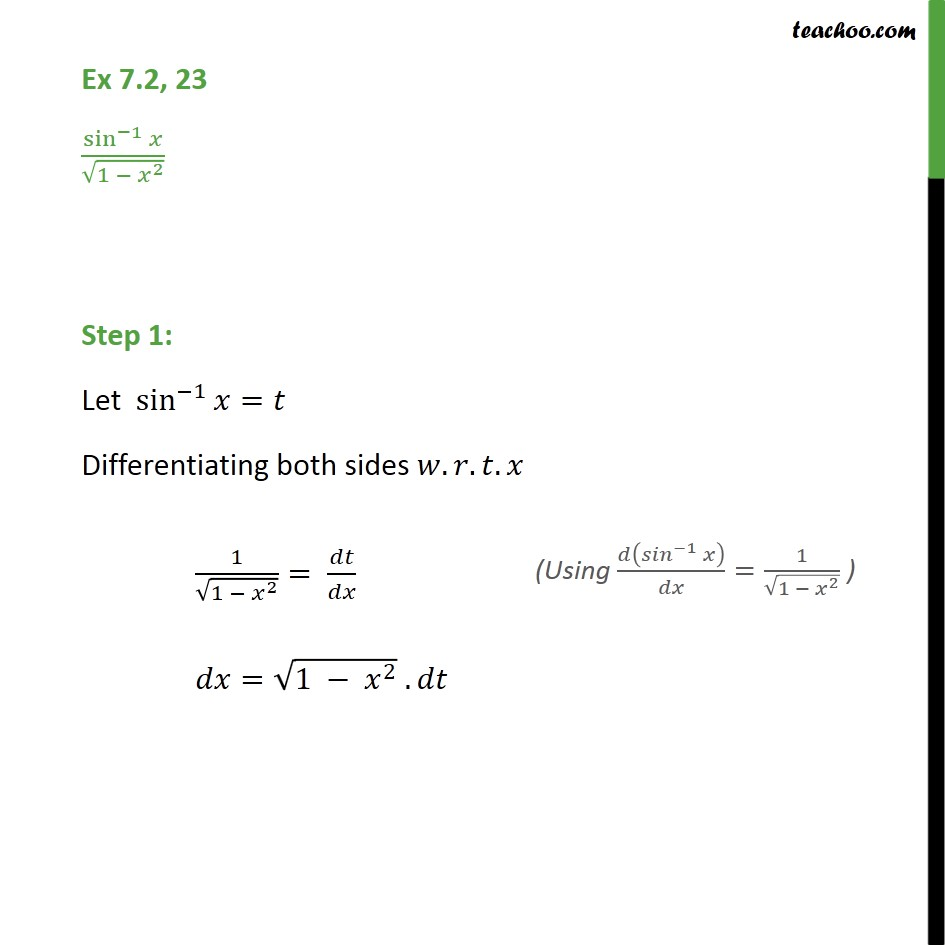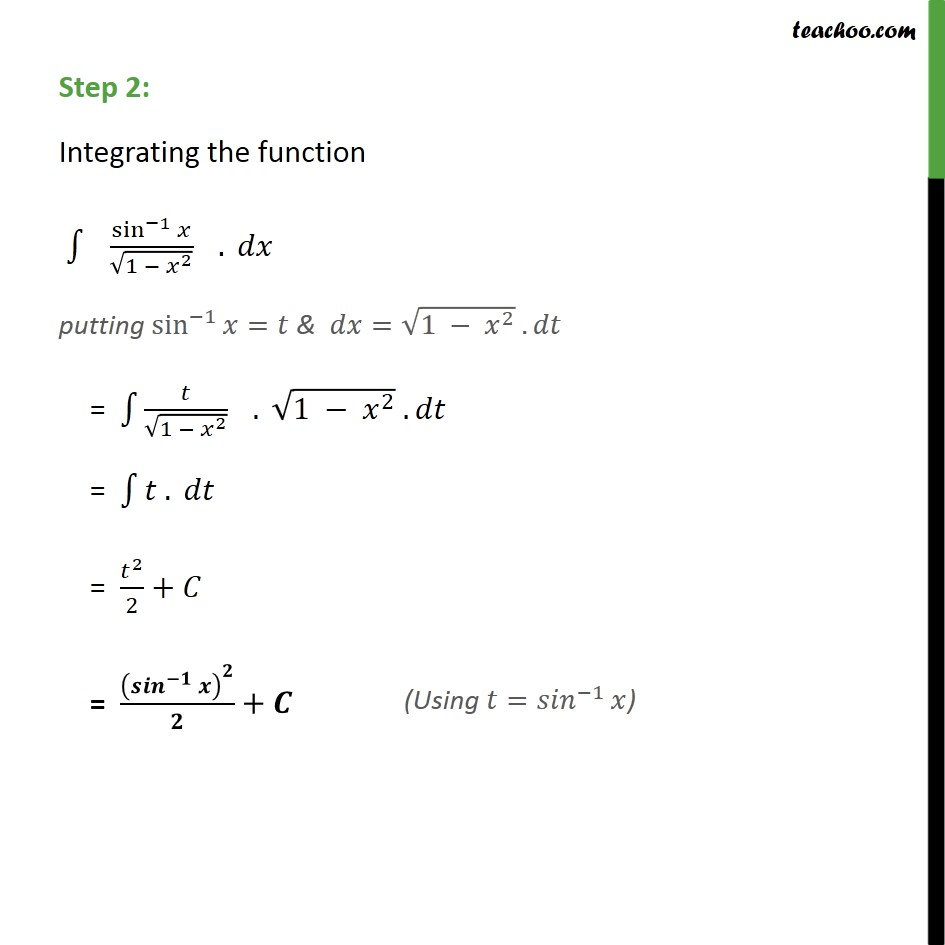Integration by substitution - Trignometric - Inverse

Chapter 7 Class 12 Integrals
Concept wiseLearn in your speed, with individual attention - Teachoo Maths 1-on-1 Class

### Transcript

Ex 7.2, 23 sin^(−1)⁡𝑥/√(1 − 𝑥^2 ) Step 1: Let sin^(−1)⁡𝑥=𝑡 Differentiating both sides 𝑤.𝑟.𝑡.𝑥 1/√(1 − 𝑥^2 )= 𝑑𝑡/𝑑𝑥 𝑑𝑥=√(1 − 𝑥^2 ) .𝑑𝑡 Step 2: Integrating the function ∫1▒〖" " sin^(−1)⁡𝑥/√(1 − 𝑥^2 ) " " 〗. 𝑑𝑥 putting sin^(−1)⁡𝑥=𝑡 & 𝑑𝑥=√(1 − 𝑥^2 ) .𝑑𝑡 = ∫1▒〖𝑡/√(1 − 𝑥^2 ) " " 〗. √(1 − 𝑥^2 ) .𝑑𝑡 = ∫1▒〖𝑡 . 𝑑𝑡〗 = 𝑡^2/2+𝐶 = (〖𝒔𝒊𝒏〗^(−𝟏)⁡𝒙 )^𝟐/𝟐+𝑪